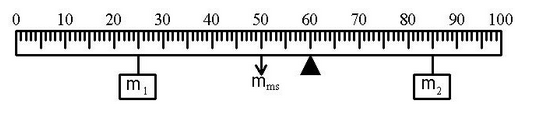# Problem: 1. The center of mass of a 0.40 kg (non-uniform) meter stick is located at its 47-cm mark. What is the magnitude of the torque (in N•m) due to gravity if it is supported at the 30-cm mark? (Use g = 9.79 m/s2).2. Suppose that a meter stick is balanced at its center. A 0.18 kg mass is then positioned at the 8-cm mark. At what cm mark must a 0.22 kg mass be placed to balance the 0.18 kg mass?3. Given the situation in the figure. The mass m1 is 0.70 kg and it is located at x1 = 25 cm. The pivot point is represented by the solid triangle located at x = 60 cm. The mass of the meter stick (mms = 0.40 kg) is located at its geometric center, xms = 50 cm. The mass m2 is 0.34 kg and it is located at x2 = 85 cm. Calculate the net torque about the pivot (in N•m with the proper sign) due to these three weights. Use g = 9.79 m/s2. Do not include units with the answer. 4. True/Fale -- The apparatus described in the previous question is in equilibrium.

###### FREE Expert Solution

1.

We are asked to determine the torque due to weight.

Torque:

$\overline{){\mathbit{\tau }}{\mathbf{=}}{\mathbit{r}}{\mathbit{F}}}$###### Problem Details

1. The center of mass of a 0.40 kg (non-uniform) meter stick is located at its 47-cm mark. What is the magnitude of the torque (in N•m) due to gravity if it is supported at the 30-cm mark? (Use g = 9.79 m/s2).

2. Suppose that a meter stick is balanced at its center. A 0.18 kg mass is then positioned at the 8-cm mark. At what cm mark must a 0.22 kg mass be placed to balance the 0.18 kg mass?

3. Given the situation in the figure. The mass m1 is 0.70 kg and it is located at x1 = 25 cm. The pivot point is represented by the solid triangle located at x = 60 cm. The mass of the meter stick (mms = 0.40 kg) is located at its geometric center, xms = 50 cm. The mass m2 is 0.34 kg and it is located at x2 = 85 cm. Calculate the net torque about the pivot (in N•m with the proper sign) due to these three weights. Use g = 9.79 m/s2. Do not include units with the answer.4. True/Fale -- The apparatus described in the previous question is in equilibrium.